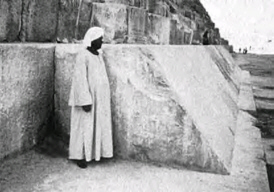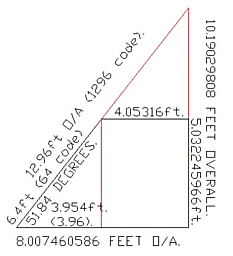The Works of Jerry Iuliano  Ancient Numbers Revealed in Scientific Formulas   Compiled by Joseph E. Mason

Cheops Pyramid Face Angle

The Cheops pyramid face angle . . . 51.85 degrees...can describe both the fine-structure constant . . . aem = 1/137.036 . . . and the Feigenbaum delta constant . . . Fd = 4.669201609...because of the ability for angle transformation that the fine-structure constant does on the face angle: transforming the face angle from degrees Sumerian..360 . . .  to radians ...Pi form. . . :

 aem = fine-structure constant = 1/137.036001 . . .   cosine in radians  Cheops pyramid face angle = 51.849982814 . . . degrees Sumerian   ( cos 51.849982814 ) * ( 10 ^ 4 ) = - 1/137.036001 . . . = 1/aem

. . . to arrive at the Feigenbaum delta constant, one must subtract the radian from the Cheops face angle. This is interesting because now the direct connection can be shown as a simple subtraction of the radian...180/pi.. from the face angle, which suggests dimensionlessness.

 (( radian - 51.849982814 ) ^ ( 1/4 )) + Pi = Fd = 4.669212726 . . .

. . . a form measured in radian degree equals the Feigenbaum delta constant . . . 4.669201609 . . . this is a complete crossover of dimensionals. Another angular crossover is the equivalency:

 ( 82944 ^ (( tan 51.85397402 ) 4 )) / 100 = cos 1/aem

. . . here tangent is measured in degrees, cosine in radians. This is the derivative:

 ( 82944 ^ ( 1/ Pi )) / 100 = cos 137.036000986

. . . subtracting modules of Beta ( .37 ) from the Cheops face angle shows several integers modulo to
( 10 ^ 2 ) residue 5.:

 51.85 - ( .37 * 5 ) = 50 51.85 - ( .37 * 105 ) = 13  51.85 - ( .37 * ( 105 + 100 ) = -24   51.85 + ( .37 * 95 ) = 87

. . . the Fermat form...e^(Pi+8) . . . generates from the Cheops face angle using Beta ( .37 ):

 ( sqrt ( e ^ ( Pi + 8 ))) / ( .37 ^ 3 ) = 51.85145318 degrees

. . . taking the Cheops face angle directly to 1/Pi power results in the strange connection to the  Hebrew  32 paths  of wisdom or  the Hindu 32 smokes of the reality.

 (( 51.849982814 ^ ( 1/ Pi )) - 3.199999974 ) * 10 = Pi

J.Iuliano

_________________

THE SIGNIFICANCE OF THE GREAT PYRAMID'S SIDE ANGLE.

Various degree angles have been postulated as the exact angle for the diagonal faces of the Great Pyramid and it is now generally accepted that the intended angle was very close to 51 degrees & 51 minutes. In a decimal rendition, this would equate to 51.85 degrees. The means whereby the angle has been estimated in modern times is based upon the few remaining weather worn casing stones at the base of the pyramid. Thankfully, one solitary course was buried under a high bank of sand during the era when the outer casing stones were being ravaged and plundered to build the city of CairoFigure 4: The few solitary casing stones at the bottom of the Great Pyramid. These too would have been removed were it not for the good fortune that they were covered in tons of sand and inaccessible to those who plundered the Pyramid's stone to build the city of Cairo. These beautiful white limestone casing stones once sheathed the entirety of the Great Pyramid on every face, all the way up to its 44' X 44' flat floor altar on the top of the pyramid.

There are various degree angles given, all in the vicinity of 51.85 degrees, which different scientists have determined represent the intended, finite angle of the Great Pyramid.

Because of the weather worn and sand lashed condition of these limestone sheathed surfaces, the exact degree angle will never be resolved, definitively, by averaging the damaged angles of the casing stones themselves. We do, however, know that the architects were aiming for a figure around 51.85 degrees.

Happily, the true figure can easily be identified mathematically, as there is only one plausible possibility.

1. The sought after, coded angle was 51.84 degrees...for the following reasons:

2. The number 51.84 is an exact fraction of 25920, the anciently used number that described, in years, the duration of the Precession of the Equinoxes for a complete circuit (51.84 X 500 = 25920).

3. The angle, 51.84 degrees, divided by 2 = 25.92...again coding the appropriate numbers related to the Precession of the Equinoxes.

4. It has been determined, through comparative analysis, that the ancient reed measurement was 10.5 feet or 126 inches. Therefore, given that the true length of the Great Pyramid was 756 feet, which equates to 72 reeds per side, the total area that the pyramid covers is 72 X 72 reeds or 5184 square reeds.

5. It will be shown that the Heel stone at Stonehenge resides 259.20 feet from the exact centre of the site to the outlying face of the stone, The azimuth angle from site centre to the south-western extremity face of the stone was intended to convey 51.84 degrees off North.Figure 5: A drawing of a surviving casing stone as per the close proximity dimensions given by W. F. Petrie. The extended lines (red) indicate the probable geometric coding that the architects wished to demonstrate in a block, 8' X 12' of base measure and having a rear height of essentially 5 feet. The 6.4 feet of diagonal face alludes to the very important "64" code. If the block were allowed to run to a point where the face diagonal intersected with the rear vertical line, then the distance covered would be 12.96 feet. This number is a very important code of antiquity that relates to the duration of the Precession of the Equinoxes . . . 25920 years / 2 = 12960 years.

. . . end of article . . .

______________

Discovered 4-19-05 . . .

Concerning the 44 foot by 44 foot altar floor at the top of the pyramid, this equation was noted: e = natural log = 2.718281828 . . .
 ((( 44.00016823 / 37 ) ^ 4 ) * 10 ) + Pi = e ^ Pi  440 / 37 = 11.891891891 . . . a collective unconscious modulo to 37

. . . concerning the angle of the face wall of the Cheops pyramid ..51.85 degrees . . . which is noted in sumerian degrees . . . 360 units...it was discovered that the fine-structure constant . . . aem = 1/137.036 . . . transformed the Cheops face angle from degrees to  radians:cos in radians

 ( cos 51.849982814 ) * 1000 = -137.036001....= -1/aem

25920 is Plato's cyclic and is a collective unconscious constant determining fine-structure:

 ( 10 ^ ( 2591.999784 / 666 ) / 666 = sqrt 137.036001

J.Iuliano

(Sent via e-mail April 23, 2005)

_______________

________________________________________________________________

Note from Joseph E. Mason:

The number 82944 is 288 squared. For more information about these and other similar numbers and their connections to ancient numbering systems, see the links on the index page.

The 44' x 44' flat floor altar on the top of the pyramid is interesting in relation to another measure:

44 x 44 = 1936
1936 = 11 x 11 x 16
1936 / 11 = 176
1760 is one mile in yards

The sought after, coded angle of 51.84 degrees, and its relation to the total area that the pyramid covers, 72 x 72 reeds or 5184 square reeds, brings forward yet another ancient number, listed on our Code of the Ancients page:

5184 = Victory over the beast (Rev. 15:2)

According to Kabbalah, there are 72 Divine Names.

It may also be significant that 4 x 72 = 288, and that 82944 / 72 = 1152, "Witness."

Some say there were originally 144,000 casing stones on the Great Pyramid. See:

The fine-structure constant is said to be the "coupling constant" or measure of the strength of the electromagnetic force that governs how electrically charged elementary particles (e.g., electron, muon) and light (photons) interact. Some say it represents the probability that an electron will emit or absorb a photon.

electron = .510998902 mev / (c ^ 2 )
Me = electron mass = 9.1093826 * ( 10 ^ - 11 ) grams
mev
= one million electron volts
Re = electron radius = 2.817940285 * ( 10 ^ - 7 ) meters
K = coulumbs constant = 8.987551705 * ( 10 ^ 9 )
Gn = gravitational constant in newtons = 6.6742 * ( 10 ^ - 11 ) k^3/m/s
c = speed of light = 299792458 meters/seconds
h = Planck's constant = 6.62606891 * ( 10 ^ - 34 ) Joules
E = permittivity of space = 8.854187818 * ( 10 ^ - 12 ) Fm<

_________________________________